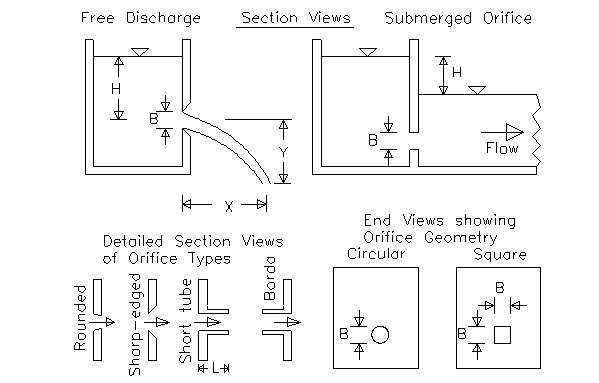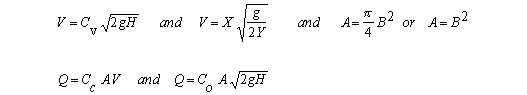Water Flowing (Discharging) Steadily from a Tank

Calculation and equations

Register to enable "Calculate" button.

 Calculate: Q from H, B, type Q, H from B, X, Y, type H from Q, B, type H from X, Y, type H, B from Q, X, Y, type H, X from Q, B, Y, type H, Y from Q, B, X, type B from Q, H, type X from H, Y, type Y from H, X, type Co from Q, H, B Cv from H, X, Y Cc, Cv, Co from Q, H, B, X, Y Discharge, Q (cfs, ft3/s): Head, H (ft): Select Units: Orifice Diameter, B (inch): English Units. Q in cfs English Units. Q in gpm Metric Units Contraction Coefficient, Cc: Velocity Coefficient, Cv: Select Orifice Geometry: Orifice Coefficient, Co: Circular. A=(PI) B2/4 Square. A=B2 Horizontal Distance, X (ft): Vertical Drop, Y (ft): Select Type of Orifice: Orifice Area, A (ft2): Rounded Orifice Edges Sharp-Edged Orifice Short Tube Re-entrant (Borda) Computed or Entered by user Discharge Velocity, V (ft/s): http://www.LMNOeng.com © 2014 LMNO Engineering, Research, and Software, Ltd.

Units in Steady State Tank Discharge calculator: cm=centimeter, ft=foot, gal=U.S. gallon, m=meter, min=minute, s=second.Either the free discharge orifice or the submerged orifice can be modeled. The equations are the same for both cases. A drop-down menu allows you to select circular or square orifice geometry. For a circular orifice, B is orifice diameter. For a square orifice, B is orifice width and height. A drop-down menu allows you to select an orifice type. Discharge coefficients for the four orifice types are built into the calculation. User-defined discharge coefficients are permitted but be sure that Co= CcCv. The calculation only checks to see if inputs are positive; we do not check to see if Co= CcCv.

Built-in values for orifice discharge coefficients

Rounded         Sharp-edged         Short tube             Borda
Cc        1.0                     0.62                     1.0                 0.52
Cv        0.98                   0.98                     0.8                 0.98

The short tube C values are valid for L ~ 2.5 B.
The Borda type is also known as a re-entrant since it juts into the tank.
C values were obtained from Dally et al. (1993) for circular orifices. However, similar values for square orifices are given in Davis (1942), so our calculation uses the Dally values for both circular and square orifices.

Equations.  From Dally et al. (1993) and Streeter et al. (1985)
The tank discharge equations are valid for:   H > 1.25 m (4.17 ft)  and  B > 2.54 cm (1 inch). Values will be computed even if H or B is too small, but a warning message will appear.where:  A = orifice area, B = orifice diameter or width and height, Cc = contraction coefficient, Cv = velocity coefficient, Co = orifice coefficient = CcCv, g = acceleration due to gravity = 32.174 ft/s2 or 9.8066 m/s2, H = head defined in diagrams above, Q = flow rate (discharge), V = horizontal velocity through orifice, X = horizontal trajectory, Y = vertical trajectory.

References

Dally, J. W., W. F. Riley, and K. G. McConnell. 1993. Instrumentation for Engineering Measurements. John Wiley and Sons, Inc. 2ed.

Davis, C. V. 1942. Handbook of Applied Hydraulics. McGraw-Hill Book Co.

Streeter, V. L., E. G. Wylie, and K. W. Bedford. 1985. Fluid Mechanics. McGraw-Hill.  9ed.

LMNO Engineering, Research, and Software, Ltd.
7860 Angel Ridge Rd.   Athens, Ohio 45701  USA   Phone: (740) 592-1890
LMNO@LMNOeng.com    http://www.LMNOeng.com

To: